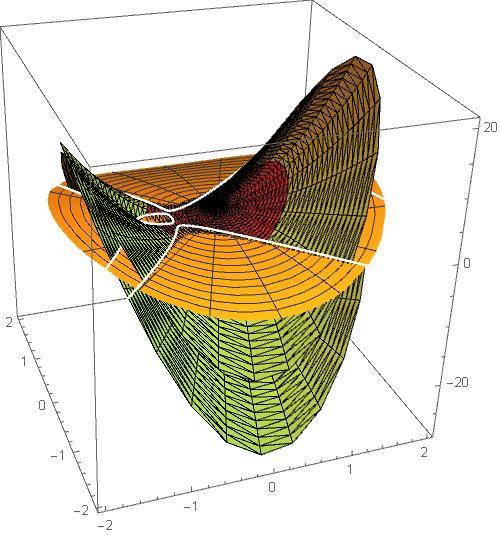# Finding intersection of two algebraic curves

• I
aheight
TL;DR Summary
Is there a standard numeric approach to finding the intersection of two algebraic curves?
Given two algebraic curves:

##f_1(z,w)=a_0(z)+a_1(z)w+\cdots+a_n(z)w^n=0##
##f_2(z,w)=b_0(z)+b_1(z)w+\cdots+b_k(z)w^k=0##

Is there a general, numeric approach to finding where the first curve ##w_1(z)## intersects the second curve ##w_2(z)##? I know for low degree like quadratic or cubics can find the intersection by brute force but was wondering if there is a more general approach for higher degrees say 10 or 12 each?

Mentor
Not expressed with radicals, at least.
If there would be then quintic equations would have such a solution. They do not in general: Abel-Ruffini theorem

•aheight
aheight
Not expressed with radicals, at least.
If there would be then quintic equations would have such a solution. They do not in general: Abel-Ruffini theorem

It appears to be a difficult problem even numerically. Was just wondering how others might approach it (numerically).

Gold Member
@aheight -- You should look at the resultant of the two polynomials. See the Resultant - Wikipedia article for more information, especially the section on Algebraic Geometry. HTH

•mathwonk
aheight
@aheight -- You should look at the resultant of the two polynomials. See the Resultant - Wikipedia article for more information, especially the section on Algebraic Geometry. HTH
Thanks for that. However, perhaps I should have stated above I'm interested in computing the intersections of the real and imaginary sheets of both algebraic curves. Consider a simple case I'm working on:

\begin{align*} f1(z,w)&=(-6z/5-2z^2+z^4)+(2/5-2 z^2/5)w+1/25 w^2=0\\ f2(z,w)&=(-1+z^2)-6/5 w=0 \end{align*}
If I plot the real sheets of ##w_1## from f1 as the yellow and red surfaces and the real sheet of ##w_2## from f2 as the orange surface in the plot below, I obtain their intersections as the white curves. The white curves I computed by solving simple simultaneous equations for this simple case which I would not be able to do with higher degree curves and was wondering if there is a systematic way to find the intersections for the higher degree cases.

Guess I mean I don't see how the resultant can be used to find the white curves.Last edited: